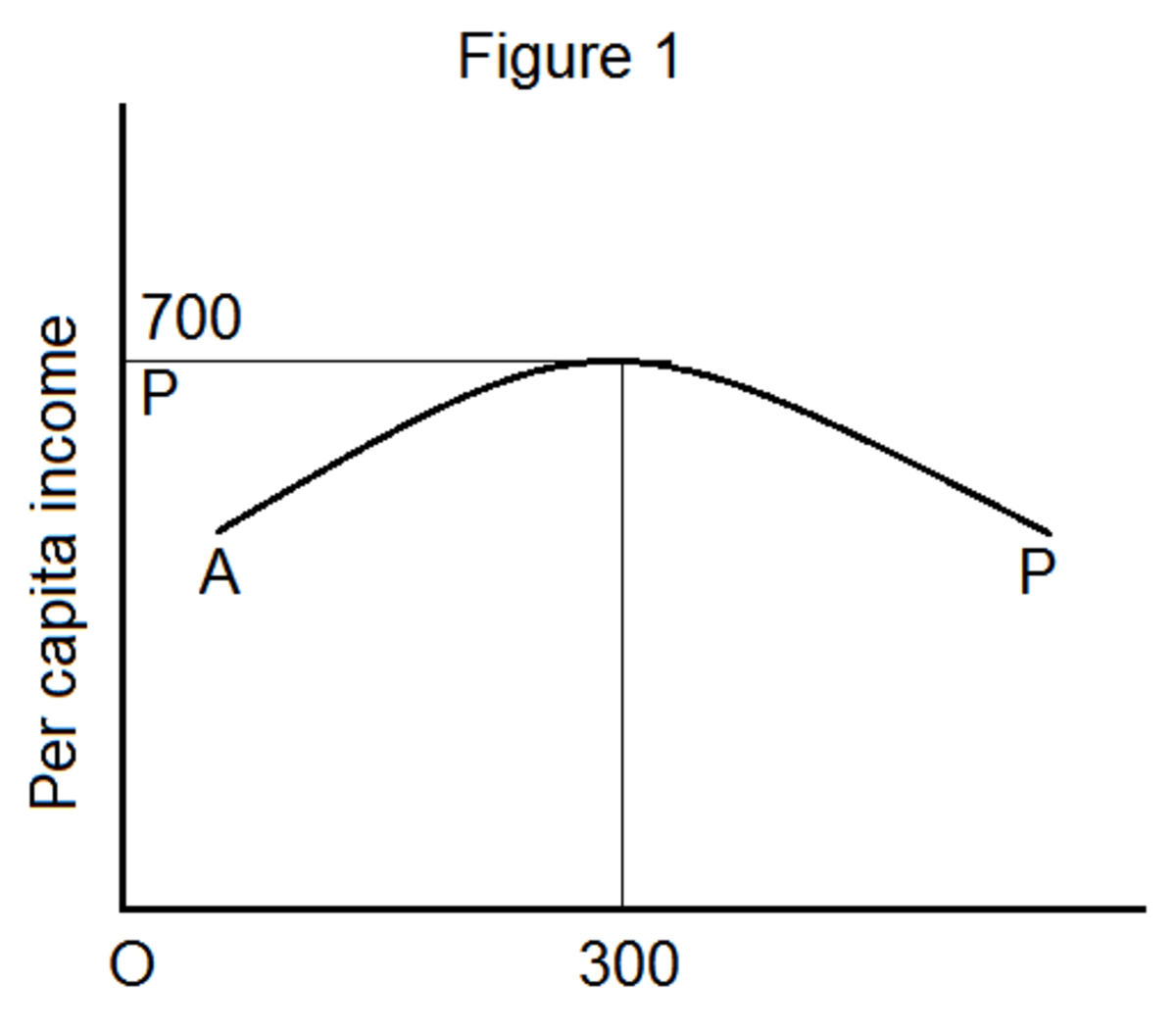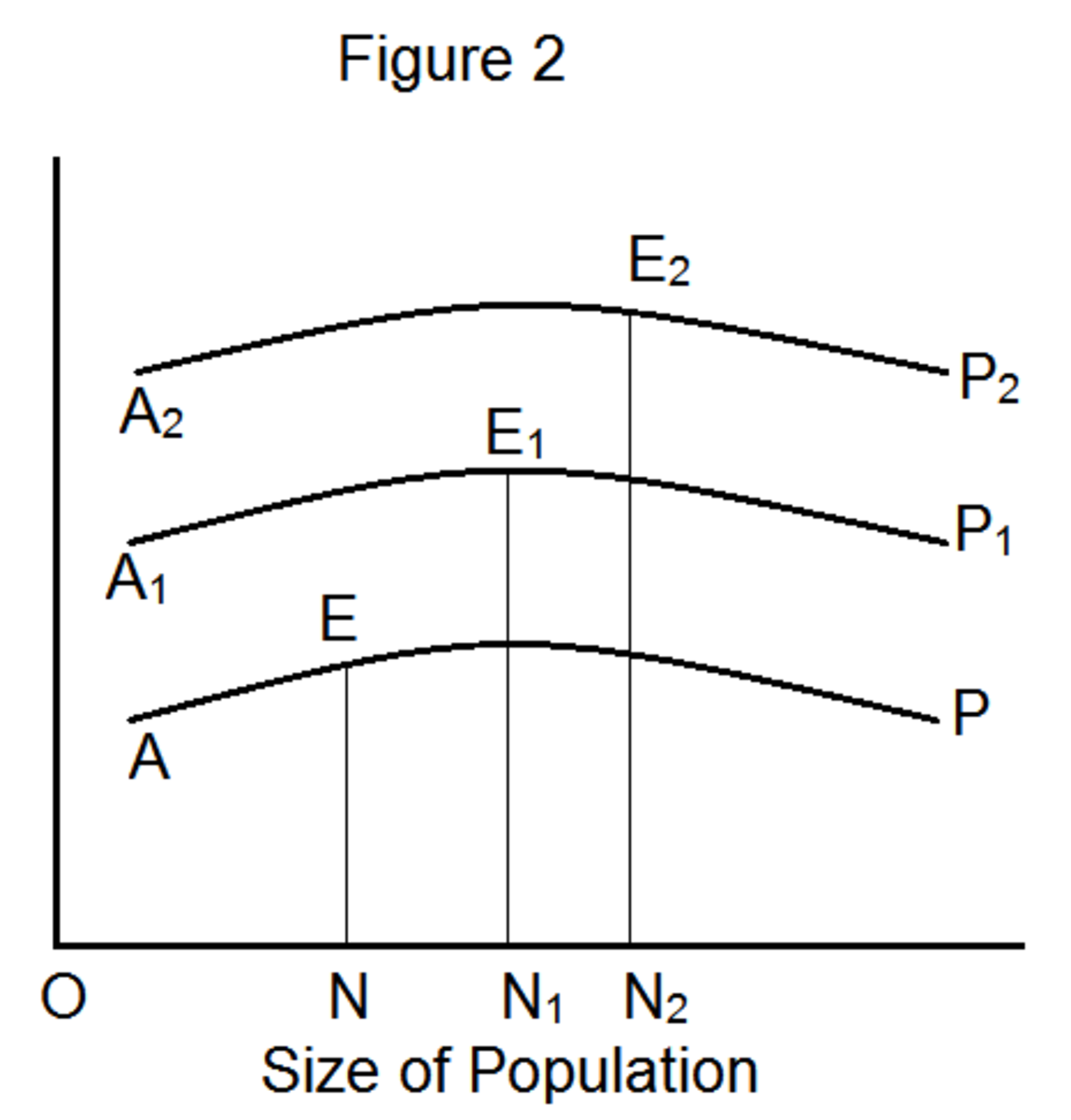Updated date:

# Optimum Population Theory

## Introduction

Many economists developed the optimum population theory. Notable economists such as Dalton, Robbins, West and Cannan contributed to the optimum population theory in a significant manner. Prof. Sidgwick was the first one, who gave hints on the optimum population theory in his reputed book, “Principles of Political Economy”. However, the book “World Population” by Carr, Saunders popularized the concept of optimum population.

Malthusian theory of population claims that if population of a country keeps on increasing, it is going to be detrimental to the society. However, optimum population theory denies this argument. Hence, we could say that the optimum population theory is the direct result of a sharp reaction to the Malthusian theory of population.

The optimum population theory claims that population growth is beneficial if it pulls the economy towards optimum. The theory further states if population growth pushes the economy away from optimum, the increase in population is harmful to the society. Note that the theory is trying to elucidate an economically ideal size of population for a country. Hence, the theory does not say anything about why population increases and what the determinants of birth and death rates are.

What is an optimum population or an ideal population size for a country?

The optimum population is the best and the most desirable size of population, which maximizes the per capita income with given natural resources, technology and capital. Anything above the desirable size of population is harmful to the society.

The whole theory is based on the following three important concepts:

1. Under-population – anything below the optimum population or desirable size of population.

2. Over-population – anything above the optimum population or desirable size of population.

3. Optimum-population – the most desirable size of population.

## Why is Under-population not Preferred?

In general, the population of a country should be in a position to utilize its natural resources optimally. If the current population of a country is not sufficient to utilize its resources effectively, it cannot produce enough goods for increasing population. Under population leads to labor shortage and hence specialization of labor or division of labor is not possible. Note that division of labor is the best production technique to utilize all resources efficiently. What matters here is whether the current population is able to utilize the available resources efficiently or optimally. If the population is able to utilize the available resources optimally, the per capita income of the country increases. Otherwise, the country is said to be underpopulated.

## Effects of Over-population

Under population can be accepted to some extent, as it does not do much harm to the society. However, if a country is over populated, it is certainly harmful to the society. Over-population means more men share limited resources. The following are the most common problems of an over populated country:

1. The available resources are not sufficient to satisfy the people’s need. Hence, the country heavily depends on imports.
2. The average productivity of labor falls because of excessive population. The available resources are not sufficient to provide employment to all.
3. The situation of disguised unemployment is very common in an over populated country. Disguised unemployment refers to a situation in which a person is unable to get a job that is suitable to his or her qualifications.
4. Other non-economic issues such as famine, war and diseases prevail, which ultimately affects the economy of the country.

Some economists argue that the over-population can be utilized well by deploying more and more capital. However, this may harm the economy further by stimulating inflation.

## Optimum Population

In order to understand the concept of optimum population in a better manner, let us look at the following illustration. Table 1 and figure 1 show how national income and per capita income behave as total population changes.

## Table 1: Population and Per capita Income

Total Population (million)National Income (million dollars)Per capita Income (\$)

100

50,000

500

150

82,500

550

200

120,000

600

250

162,500

650

300

210,000

700

350

238,000

680

400

264,000

660

450

274,500

610

500

275,000

550

From the table given above, we can understand that national income of the society increases as population increases. However, the per capita income initially increases, reaches maximum and then starts declining. When population is 300 million, the per capita income reaches the maximum level (\$700). Hence, the optimum level of population or the most desirable size of population for the country is 300 million. Any deviation from this optimum level leads to less per capita income.Figure 1 shows the relationship between population size and per capita income. In figure 1, the horizontal axis measures the size of population and the vertical axis measures the per capita income. AP represents the per capita income curve. OP indicates the optimum level of population.

## Assumptions of the Optimum Population Theory

There are two important assumptions for the theory to hold good. They are:

i. Even though the population increases, the percentage of working population to total population remains constant.

ii. There is no change in natural resources, capital and technology. In other words, an increase in the population does not alter the available natural resources, capital and technology.

## Can optimum size of population change?

The answer is ‘yes’. The optimum population is not fixed and changes as there is a change in the factors such as available capital and technology. In our example (table 1), the optimum size of population is 300 million given the natural resources, state of technology and capital. However, these factors are subject to change. If there is a technological improvement, the optimum size of population also changes accordingly. Figure 2 explains this situation in a better manner.In figure 2, AP represents the original per capita income curve with ON size of population and EN per capita income. Suppose there is a change in natural resources and technology. With a change in natural resources and technology, the per capita income curve shifts to A1P1 and then further to A2P2. Consequently, the corresponding levels of optimum population are ON1 and ON2 respectively.

According to Dr. Dalton, the deviation of actual population from the optimum population is known as maladjustment. Dr. Dalton has designed a formula to calculate the degree of maladjustment.

The formula to calculate the maladjustment is:

M = (A-O) / O

Where,

M = Degree of maladjustment

A = Actual population

O = Optimum population

If M>O, it is a case of over-population.

If M<O, it is a case of under-population.

If M = O, the population is the optimum level.

Illustration:

Suppose the actual population of a country is 100 million. The optimum population is 50 million.

Then the degree of maladjustment is:

M = (100-50) / 50 = 50/50 = 1

The numerical value (1) means that the country is over-populated by 100 per cent. Note that tis formula is helpful to find only the maladjustment of deviation of actual population from the optimum population. The formula cannot find what the optimum population is.

## Optimum Population Theory versus Malthusian Theory of Population

Malthusian Theory of PopulationOptimum Population Theory

Applicable to over-populated countries only

Applicable to all countries, irrespective of their economic condition

Pessimistic in nature (worries only about the disadvantages of over-population)

Optimistic in its approach (explores both advantages and disadvantages of increasing population)

Theory states that every increase in population is bad.

The theory states that increase in population can be good or bad depending upon how the population contributes to the economy.

It talks about minimum subsistence level.

It talks about maximum per capita income.

The theory believes in the law of diminishing returns.

The theory explains how both increasing returns and decreasing returns work as population increases.

The theory is static, as it considers no changes in factors such as natural resources, technology and capital.

The theory is dynamic as it considers changes in factors such as natural resources, technology and capital.

It tries to explain the relationship between population and food supply.

It tries to explain the relationship between population and country’s wealth.

It looks at man as a consumer only.

It looks at man as both a consumer and a producer.

## Criticism of the Optimum Population Theory

Even though the optimum population theory is superior to Malthusian theory of population in many aspects, the theory possesses some drawbacks as well. They are:

The very first criticism is that it is difficult to determine the optimum size of population for a country. Though we are able to find the optimum population size, it is difficult to maintain the population size at the optimum level in the long-run. In other words, the theory fails to explain how to stabilize the employment level, income and output of the country at the optimum level.

The assumption that the percentage of working population to the total population remains constant cannot be correct in reality.

The theory is concerned only about the wealth of nation. It does not explain how the wealth is distributed among the existing population.

The theory fails to explain the quality of labor force. For instance, increasing population with low quality labor force may not be helpful to increase the per capita income.

© 2013 Sundaram Ponnusamy

## Comments

Brajabandhu Mahanta on July 09, 2014:

Comparative analysis of Malthusian population Theory and Concept of Optimum population helps us how population growth and the technological developments are inter related. It is true that population increases over time may be in a slower rate but it expands it s size but not that of our natural resources, although, by applying modern scientific knowledge we are able to discover more and more opportunities to utilize our resource and manpower but still we are lag behind to sustain our optimum level. Very good article...